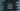# 3 different C programs to print an inverted Pyramid pattern## How to print an inverted pyramid pattern in C:

In this post, we will learn how to print an inverted pyramid pattern in C. The program will take the height of the pattern as input from the user and print the pattern using star or any other character. I will show you how to create the algorithm and how to write the program with the algorithm.

### Inverted pyramid pattern algorithm:

An inverted pyramid pattern of height 5 looks as like below:

``````* * * * *
* * * *
* * *
* *
*``````

This pattern is printed with star and blank spaces. If I replace the blank spaces with #, it will be:

``````* * * * *
#* * * *
##* * *
###* *
####*``````

There is still one blank space printed in between the stars, but we added # for the starting spaces.

You can see here,

• There are 0 starting blank spaces for the first line.
• There is 1 starting blank space for the second line.
• There are 2 starting blank spaces for the third line etc.

So, we can use three loops to print this pattern. One will be the outer loop and the other two will be the inner loops. The outer loop will point to each row of the pattern and the first inner loop will print the starting spaces and the second inner loop will print the content of the pattern.

Let’s say a variable height is the height of the pattern,

• The outer loop will run from i = 0 to i = height - 1.

• The first inner loop will run i number of times to print the blank spaces.
• The second inner loop will run for height - i number of times to print the stars. It will also add blank spaces in between the stars.
• At the end of each iteration of the outer loop, it will print one new line to move to the next line.

Let’s use this algorithm to write down the program.

### Method 1: C program to print an inverted pyramid pattern by using for loops:

Let’s use for loops to print an inverted pyramid pattern in C:

``````#include <stdio.h>

int main()
{
int height;

printf("Enter the height of the pyramid: ");
scanf("%d", &height);

for (int i = 0; i < height; i++)
{
for (int j = 0; j < i; j++)
{
printf(" ");
}

for (int j = 0; j < height - i; j++)
{
printf("* ");
}
printf("\n");
}
return 0;
}``````

In this program,

• The height is an integer variable to hold the height of the triangle. It asked the user to enter the height, read it and store it in the height variable.
• The outer for loop runs from i = 0 to i = height - 1. On each iteration of the loop,

• It runs one for loop to print the blank spaces. It runs i number of times.
• The second inner for loop prints the stars. It runs for height - i number of times.
• At the end of each iteration of the outer loop, it prints a new-line character to move the control to the next line.

If you run this program, it will give the output as below:

``````Enter the height of the pyramid: 6
* * * * * *
* * * * *
* * * *
* * *
* *
*

Enter the height of the pyramid: 7
* * * * * * *
* * * * * *
* * * * *
* * * *
* * *
* *
* ``````

### Method 2: C program to print an inverted pyramid pattern by using while loops:

We can change the for loops to while loops. Let’s write the above program using while loops:

``````#include <stdio.h>

int main()
{
int height, i = 0, j;

printf("Enter the height of the pyramid: ");
scanf("%d", &height);

while (i < height)
{
j = 0;
while (j < i)
{
printf(" ");
j++;
}

j = 0;
while (j < height - i)
{
printf("* ");
j++;
}

printf("\n");
i++;
}
return 0;
}``````

This program is using while loops to print the pyramid. It will give similar output.

### Method 3: C program to print an inverted pyramid pattern by using a separate function:

We can create a separate function to print the pattern. It will help us to separate the code to print the pattern. This function will take the height of the triangle as its parameter and it will print the pattern.

``````#include <stdio.h>

void printInvertedPyramid(int height)
{
for (int i = 0; i < height; i++)
{
for (int j = 0; j < i; j++)
{
printf(" ");
}

for (int j = 0; j < height - i; j++)
{
printf("* ");
}
printf("\n");
}
}

int main()
{
int height;

printf("Enter the height of the pyramid: ");
scanf("%d", &height);

printInvertedPyramid(height);
return 0;
}``````

Here, the printInvertedPyramid function is used to print the pattern. It uses for loops to print the pattern. It will print similar outputs.